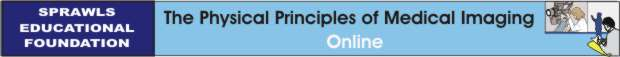Computed Tomography Image Formation
Learning Objectives

1. Identify and briefly describe the three major phases in the CT imaging process.

2. Compare CT to radiography with respect to: contrast sensitivity, visibility of detail, and spatial characteristics.

3. Explain what occurs during the scanning phase and what is produced.

4. Describe the shape and general dimensions of a typical x-ray beam used in CT.

5. Describe the two types of movement of the x-ray beam relative to the patient's body during CT imaging.

6. Explain the concept of a view as it occurs during the CT scanning process.

7. Describe how a view is divided into rays and the factors that generally determine the dimensions of a ray.

8. Describe the general type of data that is produced by one view.

9. Explain why multiple views are required to produce an image.

10. Describe the scan-and step slice acquisition method and the general characteristics of the data sets it produces.

11. Describe the helical/spiral volume acquisition method and the general characteristics of the data set it produces.

12. Explain and illustrate the concept of pitch in spiral scanning.

13. Calculate the pitch if the beam thickness is 5mm and the body is moved 10mm per tube revolution.

14. Describe the general effect of changing pitch for a scan.

15. Explain what occurs during the image reconstruction phase and what is produced.

16. Describe and illustrate the general concept of the back-projection method of image reconstruction.

17. Explain what is meant by "filtered" back projection.

18. Sketch a slice of tissue and illustrate the concept of voxels that are formed during image reconstruction.

19. Describe and illustrate the concept of pixels and their relationship to voxels.

20. Briefly describe the physical characteristic of each tissue voxel that is measured by the CT imaging process.

21. Show how the CT number for each voxel is calculated from the attenuation characteristics of the tissue.

22. Explain the significance of water with respect to CT numbers.

23. Describe and illustrate the general range of CT numbers for tissue and materials in a human body.

24. Describe what occurs during the digital-to-analog phase and what is produced.

25. Describe and illustrate the process of windowing and the effects of changing level and width.

26. Explain how windowing contributes to high contrast sensitivity.

27. Illustrate what happens when zooming is used when viewing an image.